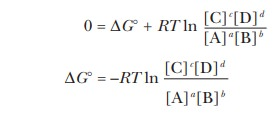## Chapter: Biochemistry: The Importance of Energy Changes and Electron Transfer in Metabolism

What are standard states? What do standard states have to do with free-energy changes?

We have already seen, how the lowering of energy, which really means dispersal on the molecular level, is spontaneous in the thermodynamic sense. In this, we are going to see how energetic considerations apply to metabolism. We are going to be comparing so many different processes that it will be useful to have a benchmark against which to make those comparisons.

## What are standard states?

We can deÞne standard conditions for any process and then use those standard conditions as the basis for comparing reactions. The choice of standard conditions is arbitrary. For a process under standard conditions, all substances involved in the reaction are in their standard states, in which case they are also said to be at unit activity. For pure solids and pure liquids, the standard state is the pure substance itself. For gases, the standard state is usually taken as a pressure of 1.00 atmosphere of that gas. For solutes, the standard state is usually taken as 1.00 molar concentration. Strictly speaking, these deÞnitions for gases and for solutes are approximations, but they are valid for all but the most exacting work.

## What do standard states have to do with free-energy changes?

For any general reaction

a A + b B -- > C + d D

we can write an equation that relates the free-energy change ( G) for the reaction under any conditions to the free-energy change under standard conditions ( ∆G°); the superscript ¡ refers to standard conditions. This equation isIn this equation, the square brackets indicate molar concentrations, R is the gas constant (8.31 J mol-1 K-1), and T is the absolute temperature. The notation ln refers to natural logarithms (to the base e) rather than logarithms to the base 10, for which the notation is log. This equation holds under all circumstances; the reaction does not have to be at equilibrium. The value of G under a given set of conditions depends on the value of ∆G° and on the concentration of reactants and products (given by the second term in the equation). Most biochemical reactions are described in terms of ∆G°, which is the G under standard conditions (1.00 M concentration for solutes). There is only one ∆G° for a reaction at a given temperature.

When the reaction is at equilibrium, ∆G = 0, and thusThe concentrations are now equilibrium concentrations, and this equation can be rewritten

∆G° = -RT ln Keq

where Keq is the equilibrium constant for the reaction. We now have a relationship between the equilibrium concentrations of reactants and products and the standard free-energy change. After we have determined the equilibrium concentrations of reactants by any convenient method, we can calculate the equilibrium constant, Keq. We can then calculate the standard free-energy change, ∆G°, from the equilibrium constant.

## Summary

Standard states are benchmark conditions chosen to have a basis of comparison for energy changes in processes under a wide variety of conditions.

Free-energy changes under standard conditions can be related to free-energy changes under any condition by a well-known equation.

Study Material, Lecturing Notes, Assignment, Reference, Wiki description explanation, brief detail
Biochemistry: The Importance of Energy Changes and Electron Transfer in Metabolism : Standard States for Free-Energy Changes |